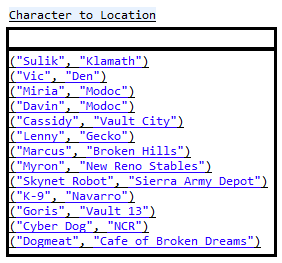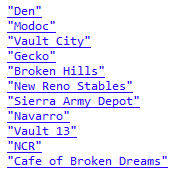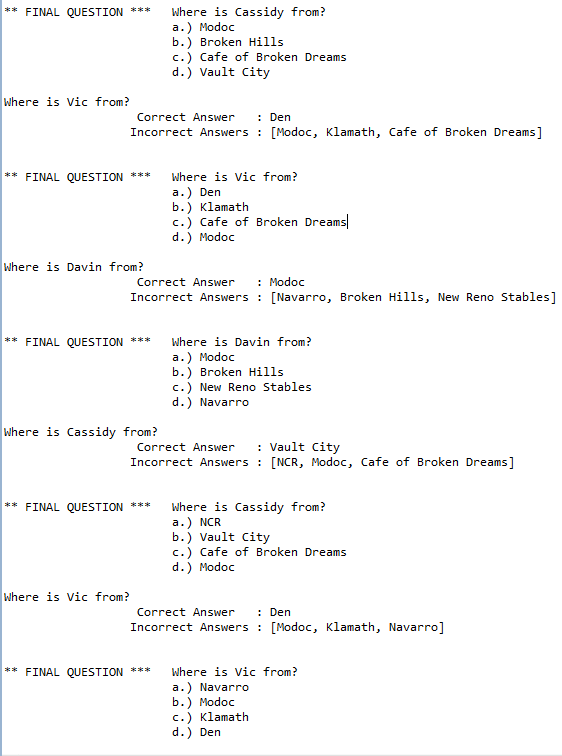# Java: LOL!: Gamification of a DataSET :D

Randomized multiple choice quiz of the relationship sets of Fallout 2 Characters and their Locations.

(Character (is from/is related to) Location)
(Concept 1 -> Relationship -> Concept 2)
Example:
( Sulik from Klamath)Now we want to ask a random question, so we get a random number between 1 and 13 , and pick that row in the data set.

We roll the dice and get “1” so we take the first data row and ask

“Where is Sulik Found?”

This quiz is going to be multiple choice of 4, so we need 3 random incorrect answers, and we need to randomize the correct answer’s location somewhere within the set of incorrect answers.

Make a List of 3 unique/distinct incorrect answers out of the remaining values within the hashtable.  Again, exclude the correct answer  and handle any duplicates.  So in Sulik‘s case, the distinct set of potential incorrect answers looks like this.Pick another random number and pick 3 distinct values from this list.  Then, append the 3 incorrect values with the correct one.  Pick another random random number 1-4 and display the randomized answers 4 times for “a.)” through “d.)“.

code for now…

```import java.util.Enumeration; import java.util.Hashtable; import java.util.LinkedList; import java.util.ListIterator; import java.util.Random;   public class QuizStructure { public static void main(String[] args) { LinkedList EveryPotentialQuestion_AnswerSet = new LinkedList();   //HOW TO MAKE A Randomized QUIZ off OF a DataSet   //Gamification of a DataSET// LOL :D   System.out.println("indeed."); Hashtable Character_to_Location = new Hashtable();   //"FALLOUT 2 Black Isle Character to Location" Relationship// //( Character - Is_From - Location )// //( Concept - Relationship - Concept ) Character_to_Location.put("Sulik", "Klamath"); Character_to_Location.put("Vic", "Den"); Character_to_Location.put("Miria", "Modoc"); Character_to_Location.put("Davin", "Modoc"); Character_to_Location.put("Cassidy", "Vault City"); Character_to_Location.put("Lenny", "Gecko"); Character_to_Location.put("Marcus", "Broken Hills"); Character_to_Location.put("Myron", "New Reno Stables"); Character_to_Location.put("Skynet Robot", "Sierra Army Depot"); Character_to_Location.put("K-9", "Navarro"); Character_to_Location.put("Goris", "Vault 13"); Character_to_Location.put("Cyber Dog", "NCR"); Character_to_Location.put("Dogmeat", "Cafe of Broken Dreams"); System.out.println(Character_to_Location.toString()); Enumeration keys = Character_to_Location.keys(); while( keys.hasMoreElements() ) { Object key = keys.nextElement(); Object value = Character_to_Location.get(key); System.out.println("Question/Answer Preview: Where is "+key+" from in Fallout 2? Answer : "+value); } Random randomGenerator = new Random(); System.out.println("size: "+Character_to_Location.size()); System.out.println("___________________________________"); //ask Ten randomized questions with 4 randomized answers for( int i = 0 ; i &lt; 10 ; i++) { int randomInt = randomGenerator.nextInt(Character_to_Location.size()); randomInt++; int iStop = 0; Enumeration morekeys = Character_to_Location.keys();   while( morekeys.hasMoreElements() ) { Object key = morekeys.nextElement(); Object value = Character_to_Location.get(key); if ( iStop == randomInt) { //System.out.println("index:" + iStop + " "+randomInt); System.out.println(); System.out.println("Where is "+key+" from?"); //Generate Multiple Choice //we know the correct answer is System.out.println(" Correct Answer : " + value); //now we need to generate other "incorrect" Choices //we need 3, Multiple Choice is Typically 4 questions LinkedList wrongAnswerList = new LinkedList(); //while wrongAnswerList is not unique //we want to remove the possibility of duplicates //we want to not include correct answer boolean is_wronglist_unique = false;   while(!is_wronglist_unique) { int randomWrong = randomGenerator.nextInt(Character_to_Location.size()); randomWrong++; Enumeration wrongkeys = Character_to_Location.keys(); int iStopInner =0; while( wrongkeys.hasMoreElements() ) { Object key2 = wrongkeys.nextElement(); Object value2 = Character_to_Location.get(key2); if ( iStopInner == randomWrong) { if ( !wrongAnswerList.contains(value2)) { if(!value2.equals(value)) wrongAnswerList.add(value2); }   } iStopInner++; } if (wrongAnswerList.size() ==3) {is_wronglist_unique = true;} } System.out.println(" Incorrect Answers : "+wrongAnswerList.toString()); System.out.println(); wrongAnswerList.add(value); System.out.println(); String [] AnswerSet = (String[]) wrongAnswerList.toArray(new String); //get 4 random ints :D //0-3 LinkedList numberset = new LinkedList(); boolean isnumbersetunique = false; int deerpCount = 0; while(!isnumbersetunique) { int randomfinal4 = randomGenerator.nextInt(4); if (!numberset.contains(randomfinal4)) { numberset.add(randomfinal4); deerpCount++; } if(deerpCount == 4) { isnumbersetunique = true; String individualquestion =""; System.out.println("** FINAL QUESTION *** Where is "+key+" from?"); individualquestion += "** FINAL QUESTION *** Where is "+key+" from?"; ListIterator itr = numberset.listIterator(); String[] abcd = {"a.)","b.)","c.)","d.)"}; int ix = 0; while(itr.hasNext()) { int get =(int) itr.next(); System.out.println(" " +abcd[ix]+" "+ AnswerSet[get]); individualquestion += " " +abcd[ix]+" "+ AnswerSet[get]; ix++; }   if ( !EveryPotentialQuestion_AnswerSet.contains(individualquestion)) { EveryPotentialQuestion_AnswerSet.add(individualquestion); //how do I know when this ^ is full ?????? ??????? //What is the most optimal way to answer this ^ without doing it manually? } } } wrongAnswerList.clear(); } iStop++; } } //the more questions you ask the more answers you'll get } }```

Output Example:What is the total number of distinct Questions and Possible combinations of Answers?  How many unique questions could be asked? Submit your answers below :D

## One thought on “Java: LOL!: Gamification of a DataSET :D”

1.mantascode

34,560 That’s what I get.